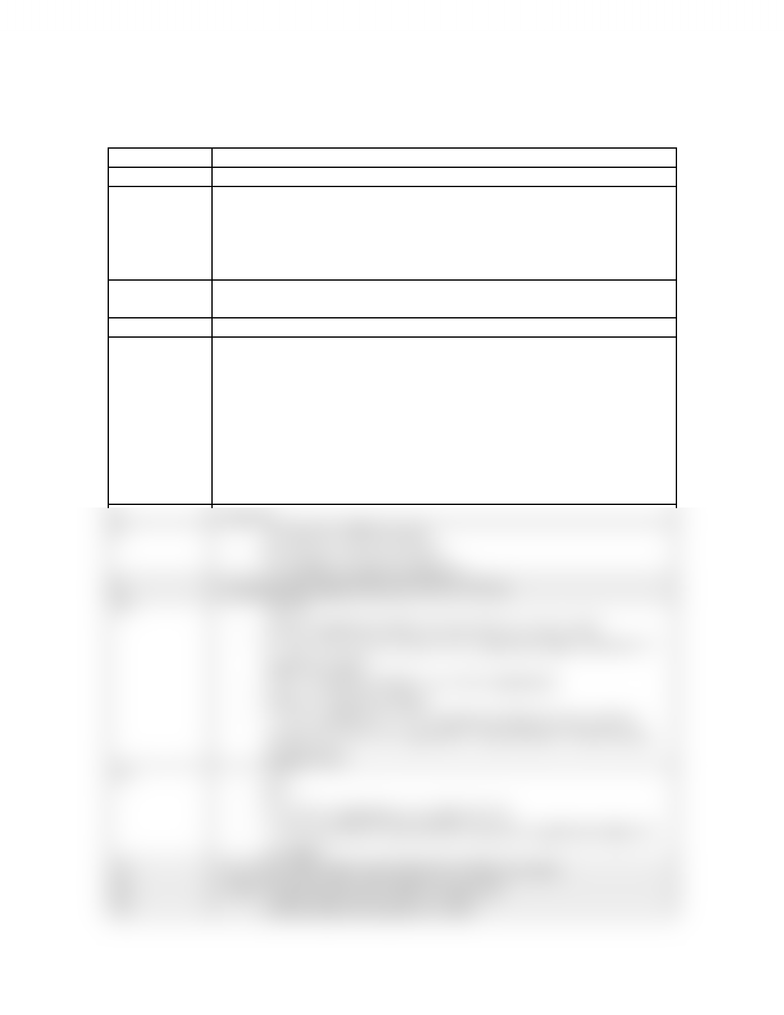Class Notes (1,100,000)
CA (640,000)
UTSG (50,000)
CHM (800)
CHM135H1 (400)
Lecture

# CHM135H1 Lecture Notes - Iodine Monochloride, Sodium Bicarbonate, Sodium Carbonate

Department
Chemistry
Course Code
CHM135H1
Professor
Kris Quinlan

This preview shows half of the first page. to view the full 2 pages of the document.LECTURE 1 – Chapters 1 & 2
Slide NumberNotes
2- Units matter
3-before SI units, units were body-derived (ie. feet)
-SI units allow for worldwide standardization, so scientists can
communicate without misunderstandings
-One-tenth of a meter is a decimetre
-One-tenth of a decimetre is a centimetre
4- must be comfortable with scientific notation, and able to use it on a
calculator
5- must know how to convert between the multiples
6-(x unit B / y unit A) is the conversation factor
-the equation gets you the measurement in unit B
-the two “unit As cancel out
-EX. 24hr(60min/1hr)(2L/1min)(1dm³/1L)
(0.1m/1dm)³(30micrograms/m³) = 86.4 micrograms
-Note that in the above equation, all units cancel out EXCEPT for
micrograms
-Formal solution to EX: Any mass above 86.4 micrograms
violates the standard.
7- Ans: D
8-A is precise AND accurate
-B is precise, but not accurate
-C is neither accurate nor precise
9- measurement ranges between 2.72 to 2.74 cm
10 -Ans: B
-A has 4 significant digits, because the two zeros count
-B – the “0.0” do not count as two significant digits, therefore 5
significant digits
-C has 2 significant digits, see A for explanation
-D has six significant digits
-*** E is ambiguous: 2 to 5 significant digits because unclear
whether the zeros are significant or placeholders (which means
insignificant)
12 -A: 5
-B: 5
-C 3-5 (for explanation, see slide 10’s E)
-***D is an EXACT QUANTITY therefore significant digits do
not apply
13 - ice is less dense than water therefore it floats on water
14 - Ans: C between the cm³ is able to cancel out
15 -another physical property is smell
www.notesolution.com